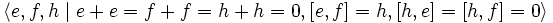# Special linear Lie ring:sl(2,2)

View a complete list of particular Lie rings

## Definition

This Lie ring can be defined in the following equivalent ways:$\langle e,f,h \mid e + e = f + f = h + h = 0, [e,f] = h, [h,e] = [h,f] = 0 \rangle$

• It is the Lie ring of strictly upper-triangular$3 \times 3$ matrices over the field of two elements, with the Lie bracket given by the commutator of two matrices$[A,B] := AB - BA$.

## Arithmetic functions

Function Value Explanation
order of a Lie ring 8
nilpotency class of a Lie ring 2
derived length of a Lie ring 2
Frattini length of a Lie ring 2

## Lie ring properties

Property Satisfied Explanation
abelian Lie ring No
nilpotent Lie ring Yes
solvable Lie ring Yes
simple Lie ring No